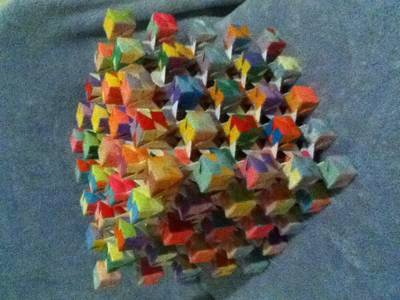# Tera Crystal Cube by Stephanie K## Tera Crystal Cube

by Stephanie K
(Oregon, USA)

The Tera Crystal Cube is made of 1,134 sheets of 2 1/8″ (5.4 cm) square paper, and 1,536 sheets of 1 1/16″ (2.7 cm) square paper, for a total of 2,670 units. The instructions for the cubes and how to connect them are in Tomoko Fuse’s book Multidimensional Transformations. It took about 150 hours to make this cube, which is 6.75″ (17 cm) high.

This is a “cube of cubes”, and I’ve found polynomial formulas that calculate the number of units needed to make one. X is the number of sub-cubes on one outer edge (in this case, 5). Total number of units used: 36x^3-90x^2+90x-30 (for this cube, it’s 2,670.) Number of 6-unit sub-cubes to make: x^3+(x-1)^3 (in this case, 189.) Number of units used in all sub-cubes: 6(x^3+(x-1)^3) (in this case, 1,134.) Number of connecting units: 24(x-1)^3 (in this case, 1,536).

Published
Categorized as Modular